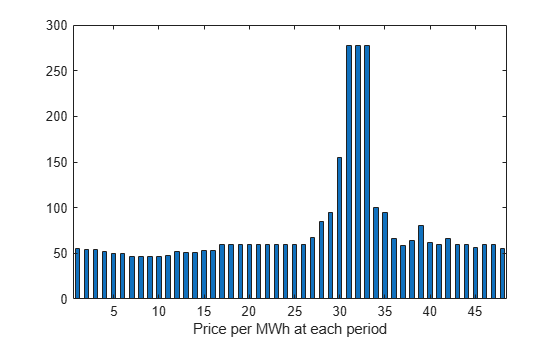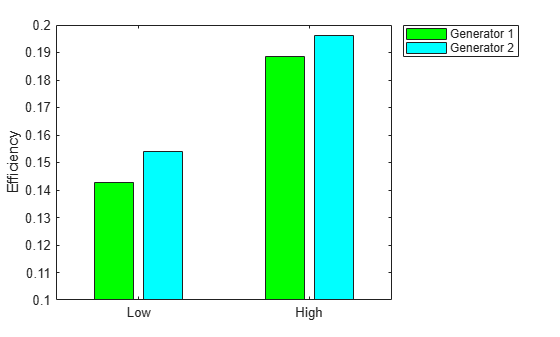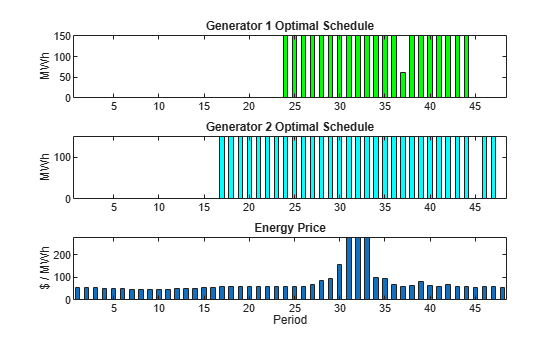Optimal Dispatch of Power Generators: Problem-Based

This example shows how to schedule two gas-fired electric generators optimally, meaning to get the most revenue minus cost. While the example is not entirely realistic, it does show how to take into account costs that depend on decision timing.

For the solver-based approach to this problem, see Optimal Dispatch of Power Generators: Solver-Based.

Problem Definition

The electricity market has different prices at different times of day. If you have generators supplying electricity, you can take advantage of this variable pricing by scheduling your generators to operate when prices are high. Suppose that you control two generators. Each generator has three power levels (off, low, and high). Each generator has a specified rate of fuel consumption and power production at each power level. Fuel consumption is 0 when the generator is off.

You can assign a power level to each generator for each half-hour time interval during a day (24 hours, so 48 intervals). Based on historical records, assume that you know the revenue per megawatt-hour (MWh) that you receive in each time interval. The data for this example is from the Australian Energy Market Operator https://www.nemweb.com.au/REPORTS/CURRENT/ in mid-2013, and is used under their terms https://www.aemo.com.au/privacy-and-legal-notices/copyright-permissions.

load dispatchPrice; % Get poolPrice, which is the revenue per MWh
bar(poolPrice,.5)
xlim([.5,48.5])
xlabel('Price per MWh at each period')There is a cost to start a generator after it has been off. Also, there is a constraint on the maximum fuel usage for the day. This constraint exists because you buy your fuel a day ahead of time, so you can use only what you just bought.

Problem Notation and Parameters

You can formulate the scheduling problem as a binary integer programming problem. Define indexes i, j, and k, and a binary scheduling vector y, as follows:

• nPeriods = the number of time periods, 48 in this case.

• i = a time period, 1 <= i <= 48.

• j = a generator index, 1 <= j <= 2 for this example.

• y(i,j,k) = 1 when period i, generator j is operating at power level k. Let low power be k = 1, and high power be k = 2. The generator is off when sum_k y(i,j,k) = 0.

Determine when a generator starts after being off. To do so, define the auxiliary binary variable z(i,j) that indicates whether to charge for turning on generator j at period i.

• z(i,j) = 1 when generator j is off at period i, but is on at period i + 1. z(i,j) = 0 otherwise. In other words, z(i,j) = 1 when sum_k y(i,j,k) = 0 and sum_k y(i+1,j,k) = 1.

You need a way to set z automatically based on the settings of y. A linear constraint below handles this setting.

You also need the parameters of the problem for costs, generation levels for each generator, consumption levels of the generators, and fuel available.

• poolPrice(i) -- Revenue in dollars per MWh in interval i

• gen(j,k) -- MW generated by generator j at power level k

• fuel(j,k) -- Fuel used by generator j at power level k

• totalFuel -- Fuel available in one day

• startCost -- Cost in dollars to start a generator after it has been off

• fuelPrice -- Cost for a unit of fuel

You got poolPrice when you executed load dispatchPrice;. Set the other parameters as follows.

fuelPrice = 3;
totalFuel = 3.95e4;
nPeriods = length(poolPrice); % 48 periods
nGens = 2; % Two generators
gen = [61,152;50,150]; % Generator 1 low = 61 MW, high = 152 MW
fuel = [427,806;325,765]; % Fuel consumption for generator 2 is low = 325, high = 765
startCost = 1e4; % Cost to start a generator after it has been off

Generator Efficiency

Examine the efficiency of the two generators at their two operating points.

efficiency = gen./fuel; % Calculate electricity per unit fuel use
rr = efficiency'; % for plotting
h = bar(rr);
h(1).FaceColor = 'g';
h(2).FaceColor = 'c';
legend(h,'Generator 1','Generator 2','Location','NorthEastOutside')
ax = gca;
ax.XTick = [1,2];
ax.XTickLabel = {'Low','High'};
ylim([.1,.2])
ylabel('Efficiency')Notice that generator 2 is a bit more efficient than generator 1 at its corresponding operating points (low and high), but generator 1 at its high operating point is more efficient than generator 2 at its low operating point.

Variables for Solution

To set up the problem, you need to encode all the problem data and constraints in problem form. The variables y(i,j,k) represent the solution of the problem, and the auxiliary variables z(i,j) indicate whether to charge for turning on a generator. y is an nPeriods-by-nGens-by-2 array, and z is an nPeriods-by-nGens array. All variables are binary.

y = optimvar('y',nPeriods,nGens,{'Low','High'},'Type','integer','LowerBound',0,...
'UpperBound',1);
z = optimvar('z',nPeriods,nGens,'Type','integer','LowerBound',0,...
'UpperBound',1);

Linear Constraints

To ensure that the power level has no more than one component equal to 1, set a linear inequality constraint.

powercons = y(:,:,'Low') + y(:,:,'High') <= 1;

The running cost per period is the cost of fuel for that period. For generator j operating at level k, the cost is fuelPrice * fuel(j,k).

Create an expression fuelUsed that accounts for all the fuel used.

yFuel = zeros(nPeriods,nGens,2);
yFuel(:,1,1) = fuel(1,1); % Fuel use of generator 1 in low setting
yFuel(:,1,2) = fuel(1,2); % Fuel use of generator 1 in high setting
yFuel(:,2,1) = fuel(2,1); % Fuel use of generator 2 in low setting
yFuel(:,2,2) = fuel(2,2); % Fuel use of generator 2 in high setting

fuelUsed = sum(sum(sum(y.*yFuel)));

The constraint is that the fuel used is no more than the fuel available.

fuelcons = fuelUsed <= totalFuel;

Set the Generator Startup Indicator Variables

How can you get the solver to set the z variables automatically to match the active/off periods of the y variables? Recall that the condition to satisfy is z(i,j) = 1 exactly when sum_k y(i,j,k) = 0 and sum_k y(i+1,j,k) = 1.

Notice that sum_k ( - y(i,j,k) + y(i+1,j,k) ) > 0 exactly when you want z(i,j) = 1.

Therefore, include these linear inequality constraints in the problem formulation.

sum_k ( - y(i,j,k) + y(i+1,j,k) ) - z(i,j) < = 0.

Also, include the z variables in the objective function cost. With the z variables in the objective function, the solver attempts to lower their values, meaning it tries to set them all equal to 0. But for those intervals when a generator turns on, the linear inequality forces z(i,j) to equal 1.

Create an auxiliary variable w that represents y(i+1,j,k) - y(i,j,k). Represent the generator startup inequality in terms of w.

w = optimexpr(nPeriods,nGens); % Allocate w
idx = 1:(nPeriods-1);
w(idx,:) = y(idx+1,:,'Low') - y(idx,:,'Low') + y(idx+1,:,'High') - y(idx,:,'High');
w(nPeriods,:) = y(1,:,'Low') - y(nPeriods,:,'Low') + y(1,:,'High') - y(nPeriods,:,'High');
switchcons = w - z <= 0;

Define Objective

The objective function includes fuel costs for running the generators, revenue from running the generators, and costs for starting the generators.

generatorlevel  = zeros(size(yFuel));
generatorlevel(:,1,1) = gen(1,1); % Fill in the levels
generatorlevel(:,1,2) = gen(1,2);
generatorlevel(:,2,1) = gen(2,1);
generatorlevel(:,2,2) = gen(2,2);

Incoming revenue = y.*generatorlevel.*poolPrice.

revenue = optimexpr(size(y));
for ii = 1:nPeriods
revenue(ii,:,:) = poolPrice(ii)*y(ii,:,:).*generatorlevel(ii,:,:);
end

The total fuel cost = fuelUsed*fuelPrice.

fuelCost = fuelUsed*fuelPrice;

The generator startup cost = z*startCost.

startingCost = z*startCost;

The profit = incoming revenue - total fuel cost - startup cost.

profit = sum(sum(sum(revenue))) - fuelCost - sum(sum(startingCost));

Solve the Problem

Create an optimization problem and include the objective and constraints.

dispatch = optimproblem('ObjectiveSense','maximize');
dispatch.Objective = profit;
dispatch.Constraints.switchcons = switchcons;
dispatch.Constraints.fuelcons = fuelcons;
dispatch.Constraints.powercons = powercons;

To save space, suppress iterative display.

options = optimoptions('intlinprog','Display','final');

Solve the problem.

[dispatchsol,fval,exitflag,output] = solve(dispatch,'options',options);
Solving problem using intlinprog.

Optimal solution found.

Intlinprog stopped because the objective value is within a gap tolerance of the
optimal value, options.AbsoluteGapTolerance = 0 (the default value). The intcon
variables are integer within tolerance, options.IntegerTolerance = 1e-05 (the
default value).

Examine the Solution

Plot the solution as a function of time.

subplot(3,1,1)
bar(dispatchsol.y(:,1,1)*gen(1,1)+dispatchsol.y(:,1,2)*gen(1,2),.5,'g')
xlim([.5,48.5])
ylabel('MWh')
title('Generator 1 Optimal Schedule','FontWeight','bold')
subplot(3,1,2)
bar(dispatchsol.y(:,2,1)*gen(1,1)+dispatchsol.y(:,2,2)*gen(1,2),.5,'c')
title('Generator 2 Optimal Schedule','FontWeight','bold')
xlim([.5,48.5])
ylabel('MWh')
subplot(3,1,3)
bar(poolPrice,.5)
xlim([.5,48.5])
title('Energy Price','FontWeight','bold')
xlabel('Period')
ylabel('\$ / MWh')Generator 2 runs longer than generator 1, which you would expect because it is more efficient. Generator 2 runs at its high power level whenever it is on. Generator 1 runs mainly at its high power level, but dips down to low power for one time unit. Each generator runs for one contiguous set of periods daily, and, therefore, incurs only one startup cost each day.

Check that the z variable is 1 for the periods when the generators start.

starttimes = find(round(dispatchsol.z) == 1); % Use round for noninteger results
[theperiod,thegenerator] = ind2sub(size(dispatchsol.z),starttimes)
theperiod = 2×1

23
16

thegenerator = 2×1

1
2

The periods when the generators start match the plots.

Compare to Lower Penalty for Startup

If you specify a lower value for startCost, the solution involves multiple generation periods.

startCost = 500; % Choose a lower penalty for starting the generators
startingCost = z*startCost;
profit = sum(sum(sum(revenue))) - fuelCost - sum(sum(startingCost));
dispatch.Objective = profit;
[dispatchsolnew,fvalnew,exitflagnew,outputnew] = solve(dispatch,'options',options);
Solving problem using intlinprog.

Optimal solution found.

Intlinprog stopped because the objective value is within a gap tolerance of the
optimal value, options.AbsoluteGapTolerance = 0 (the default value). The intcon
variables are integer within tolerance, options.IntegerTolerance = 1e-05 (the
default value).
subplot(3,1,1)
bar(dispatchsolnew.y(:,1,1)*gen(1,1)+dispatchsolnew.y(:,1,2)*gen(1,2),.5,'g')
xlim([.5,48.5])
ylabel('MWh')
title('Generator 1 Optimal Schedule','FontWeight','bold')
subplot(3,1,2)
bar(dispatchsolnew.y(:,2,1)*gen(1,1)+dispatchsolnew.y(:,2,2)*gen(1,2),.5,'c')
title('Generator 2 Optimal Schedule','FontWeight','bold')
xlim([.5,48.5])
ylabel('MWh')
subplot(3,1,3)
bar(poolPrice,.5)
xlim([.5,48.5])
title('Energy Price','FontWeight','bold')
xlabel('Period')
ylabel('\$ / MWh')starttimes = find(round(dispatchsolnew.z) == 1); % Use round for noninteger results
[theperiod,thegenerator] = ind2sub(size(dispatchsolnew.z),starttimes)
theperiod = 3×1

22
16
45

thegenerator = 3×1

1
2
2